### Home > MC2 > Chapter 10 > Lesson 10.1.5 > Problem10-64

10-64.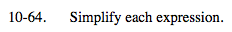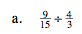When you divide by a fraction, you are multiplying by its reciprocal.

$\frac{9}{20}$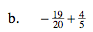Find a common denominator.

$-\frac{3}{20}$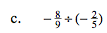See part (a).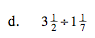Convert these mixed numbers into fractions greater than one.

$\frac{49}{16}$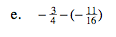${ \text{This is the same as }\\-\frac{3}{4}+\frac{11}{16}}$

$-\frac{1}{16}$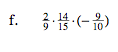${\text{This is the same as }\\\frac{2}{9}\left(-\frac{9}{10}\right)\cdot\frac{14}{15}}$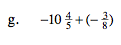You may add whole numbers and fractions separately.

$(-10)+\left(-\frac{4}{5}+-\frac{3}{8}\right)$

$-11\frac{7}{40}$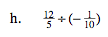See part (a).